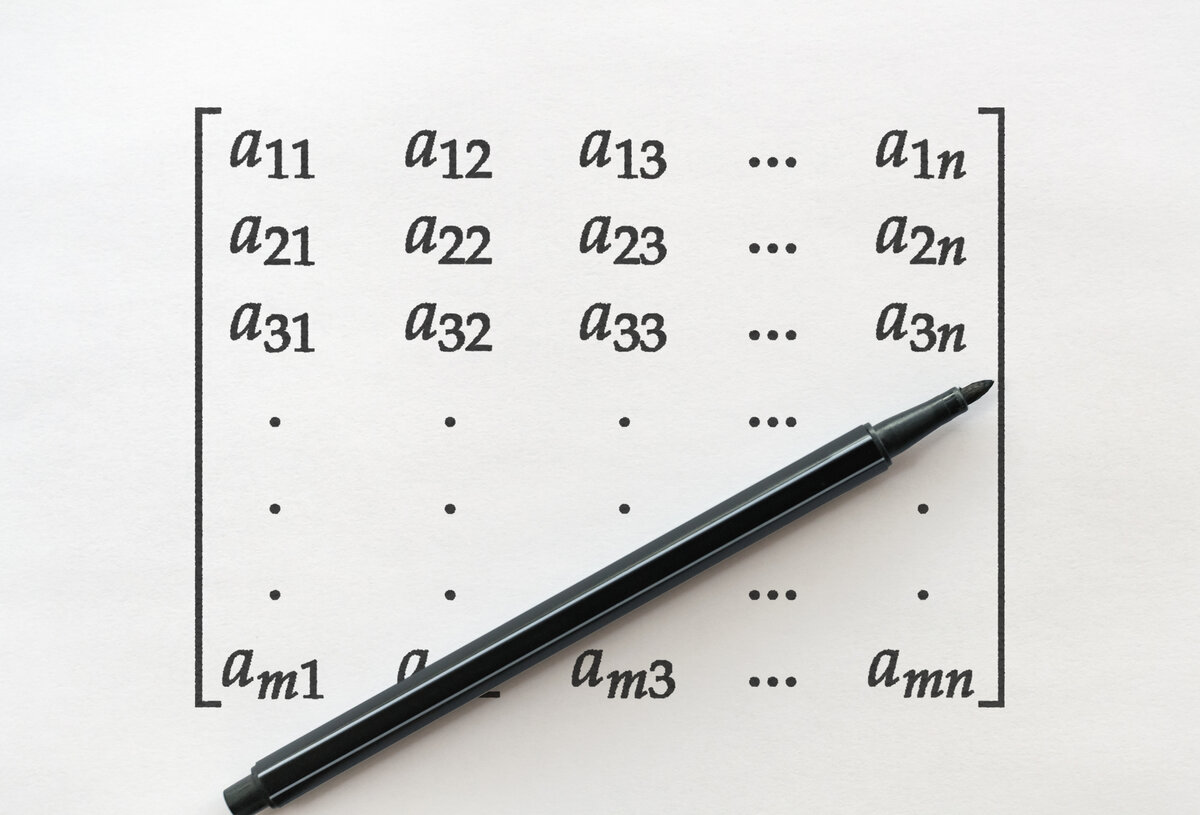# How To Create a NumPy ArrayIn this tutorial, I will show you how to create an array using the NumPy library, a scientific computing library in Python.

# Real-World Applications

• Any computer vision application written in Python that handles images or videos could use NumPy.

Let’s get started!

# Installation and Setup

We now need to make sure we have all the software packages installed. Check to see if you have OpenCV installed on your machine. If you are using Anaconda, you can type:

`conda install -c conda-forge opencv`

Alternatively, you can type:

`pip install opencv-python`

Make sure you have NumPy installed, a scientific computing library for Python.

If you’re using Anaconda, you can type:

`conda install numpy`

Alternatively, you can type:

`pip install numpy`

# Write the Code

Open up a new Python file called numpy_array_creation.py.

Here is the full code:

```# Project: How To Create a NumPy Array
# Date created: February 24, 2021
# Description: Basics of using the NumPy library

import numpy as np # Import the NumPy library

# Create and print a two dimensional array with 7 rows and 4 columns
my_array = np.zeros((7,4))
#print(my_array)

# Print the data type
#print(my_array.dtype)

# Print the dimensions of the array
#print(my_array.shape)
#print("Number of rows in the array = {}".format(my_array.shape))
#print("Number of columns in the array = {}".format(my_array.shape))

# Create an array of ones that contains 8-bit unsigned integers
my_array_ones = np.ones((7,4), dtype=np.uint8)
#print(my_array_ones)

# Create an array of random numbers
my_array_random_nums = np.random.rand(7,4)
#print(my_array_random_nums)

# Create a 4x3 two-dimensional array (i.e. a matrix)
my_2d_array = np.array([[0, 1, 2, 3],
[4, 5, 6, 7],
[8, 9, 10, 11]])

#print(my_2d_array)

# Extract the value from the matrix on row 3, column 2 (i.e. the 9)
#print(my_2d_array[2,1])
```

# Code Output

```# Create and print a two dimensional array with 7 rows and 4 columns
my_array = np.zeros((7,4))
print(my_array)
```
```# Print the data type
print(my_array.dtype)
```
```# Print the dimensions of the array
print(my_array.shape)
print("Number of rows in the array = {}".format(my_array.shape))
print("Number of columns in the array = {}".format(my_array.shape))
```
```# Create an array of ones that contains 8-bit unsigned integers
my_array_ones = np.ones((7,4), dtype=np.uint8)
print(my_array_ones)
```
```# Create an array of random numbers
my_array_random_nums = np.random.rand(7,4)
print(my_array_random_nums)
```
```# Create a 4x3 two-dimensional array (i.e. a matrix)
my_2d_array = np.array([[0, 1, 2, 3],
[4, 5, 6, 7],
[8, 9, 10, 11]])
print(my_2d_array)
```
```# Extract the value from the matrix on row 3, column 2 (i.e. the 9)
print(my_2d_array[2,1])
```

That’s it. Keep building!